Courses

# Second Law Of Thermodynamics, Entropy - MCQ Test 1

## 20 Questions MCQ Test Mock Test Series - Mechanical Engineering (ME) for GATE 2020 | Second Law Of Thermodynamics, Entropy - MCQ Test 1

Description
This mock test of Second Law Of Thermodynamics, Entropy - MCQ Test 1 for Mechanical Engineering helps you for every Mechanical Engineering entrance exam. This contains 20 Multiple Choice Questions for Mechanical Engineering Second Law Of Thermodynamics, Entropy - MCQ Test 1 (mcq) to study with solutions a complete question bank. The solved questions answers in this Second Law Of Thermodynamics, Entropy - MCQ Test 1 quiz give you a good mix of easy questions and tough questions. Mechanical Engineering students definitely take this Second Law Of Thermodynamics, Entropy - MCQ Test 1 exercise for a better result in the exam. You can find other Second Law Of Thermodynamics, Entropy - MCQ Test 1 extra questions, long questions & short questions for Mechanical Engineering on EduRev as well by searching above.
QUESTION: 1

Solution:
QUESTION: 2

### Heat transfer takes place according to

Solution:

Heat transfer takes place according to second law of thermodynamics as it tells about the direction and amount of heat flow that is possible between two reservoirs.

QUESTION: 3

### Consider the following statements:  1. Boiling of water from a heat source at the same boiling temperature. 2. Theoretical isothermal compression of a gas. 3. Theoretical polytropic compression process with heat rejection to atmosphere. 4. Diffusion of two ideal gases into each other at constant pressure and temperature. Which of these processes are irreversible?

Solution:

Any natural process carried out with a finite temperature gradient is an
irreversible process. All spontaneous processes are irreversible. Statement -4 is a spontaneous process.

QUESTION: 4

Assertion (A): Heat cannot spontaneously pass from a colder system to a hotter system without simultaneously producing other effects in the surroundings.

Reason (R): External work must be put into heat pump so that heat can be transferred from a cold to a hot body.

Solution:

A and R are true. A is the Clausius statement of second law of thermodynamics. Spontaneously means without change in surroundings. If question comes like following then answer will be (a).
Assertion (A): External work must be put into heat pump so that heat can be transferred from a cold to a hot body.
Reason (R): Heat cannot spontaneously pass from a colder system to a hotter system without simultaneously producing other effects in the surroundings.

QUESTION: 5

An inventor says that his new concept of an engine, while working between temperature limits of 27°C and 327°C rejects 45% of heat absorbed from the source. His engine is then equivalent to which one of the following engines?

Solution:

Carnot efficiency of engine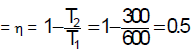But according to the inventor‟s Claim
Efficiency of engine = 1-0.45 = 0.55

∴ Efficiency of Actual Engine cannot be greater than Carnot efficiency. So this is an impossible engine

QUESTION: 6

Assertion (A): Efficiency of a reversible engine operating between temperature limits T1 and T2 is maximum.

Reason (R): Efficiency of a reversible engine is greater than that of an irreversible engine.

Solution:
QUESTION: 7

When a process becomes irreversible due to heat interaction between system and surrounding at the boundary due to finite temperature gradient, then the irreversibility is______

Solution:
QUESTION: 8

A reversible engine operates between temperatures 900 K & T2 (T2 < 900 K), & another reversible engine between T2 & 400 K (T2 > 400 K) in series. What is the value of T2 if work outputs of both the engines are equal?

Solution:

Figure from another question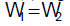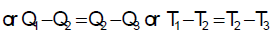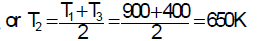QUESTION: 9

Two reversible engine operate between thermal reservoirs at 1200 K, T2K and 300 K such that 1st engine receives heat from 1200 K reservoir and rejects heat to thermal reservoir at T2K, while the 2nd engine receives heat from thermal  reservoir at T2K and rejects heat to the thermal reservoir at 300 K. The efficiency of both the engines is equal.

What is the value of temperature T2?

Solution: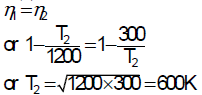QUESTION: 10

An engine working on Carnot cycle rejects 40% of absorbed heat from the source, while the sink temperature is maintained at 27°C, then what is the source temperature?

Solution:

Sink temperature = 27C = 27 + 273 = 300K
It is given that engine rejects 40% of absorbed heat from the source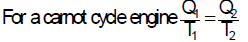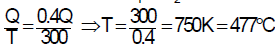QUESTION: 11

A reversible heat engine rejects 50 percent of the heat supplied during a cycle of operation. If this engine is reversed and operates as a heat pump, then what is its coefficient of performance?

Solution: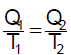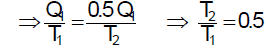If the engine is reversed and operated as the Heat Pump.

Then COP coefficient of performance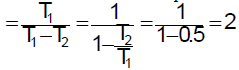QUESTION: 12

One reversible heat engine operates between 1600 K and T2 K, and another reversible heat engine operates between T2K and 400 K. If both the engines have the same heat input and output, then the temperature T2 must be equal to:

Solution:

Two reversible heat engines operate between limits of

1600K and T2; T2 and 400K

Both have the same heat input and output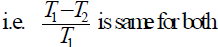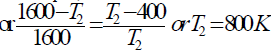QUESTION: 13

Assertion (A): No machine would continuously supply work without expenditure of some other form of energy.

Reason (R): Energy can be neither created nor destroyed, but it can only be transformed from one form into another.

Solution:
QUESTION: 14

A reversible engine En as shown in the given figure draws 300 kcal from 200 K reservoir and does 50 kcal of work during a cycle. The sum of heat interactions with the other two reservoir is given by: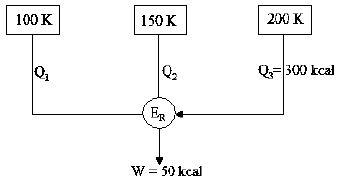Solution: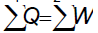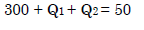QUESTION: 15

In a cyclic heat engine operating between a source temperature of 600°C and a sink temperature of 20°C, the least rate of heat rejection per kW net output of the engine is

Solution:
QUESTION: 16

An ideal gas of mass m and temperature T1 undergoes a reversible isothermal process from an initial pressure P1 to final pressure P2. The heat loss during the process is Q. The entropy change ΔS of the gas is

Solution:
QUESTION: 17

Nitrogen gas (molecular weight 28) is enclosed in a cylinder by a piston, at the initial condition of 2 bar, 298 K and 1 m3. In a particular process, the gas slowly expands under isothermal condition, until the volume becomes 2m3. Heat exchange occurs with the atmosphere at 298 K during this process.

The entropy change for the Universe during the process in kJ/K is

Solution:

It is reversible process so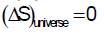QUESTION: 18

One kilogram of water at room temperature is brought into contact with a high temperature thermal reservoir. The entropy change of the universe is:

Solution:

It is a case of spontaneous process i.e. irrepressibility involved that so why entropy change of the universe is positive.

QUESTION: 19

The ratio of root mean square velocity to average velocity of gas molecules at a particular temperature is

Solution:
QUESTION: 20

Which of the following statement is correct?

Solution:

The change of entropy may be regarded as a measure of the rate of availability of heat for transformation into work.